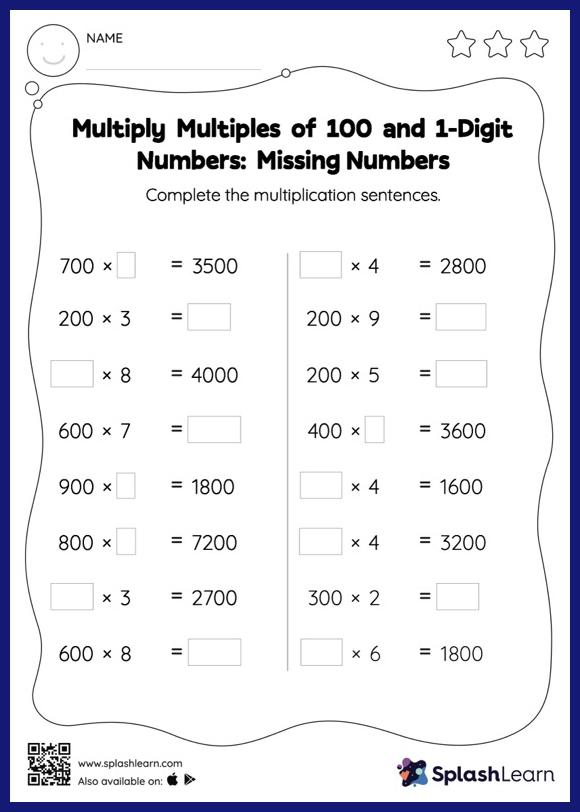# Multiply Multiples of 100 and 1-Digit Numbers: Missing Numbers Worksheet

Home > Multiply Multiples of 100 and 1-Digit Numbers: Missing NumbersThis multiply multiples of 100 and 1-digit numbers worksheet consists of a set of questions on multiplication, which help students develop fluency. When asked to multiply numbers by 10, 100, or their multiples, students use the pattern of zeros. They use the same idea to find the missing number in multiply multiples of 100 and 1-digit numbers worksheet. In each problem, the numbers are laid out in the horizontal format. Students should try to use different strategies involving composing and decomposing numbers to solve these problems. This will help them develop flexibility and fluency.